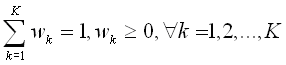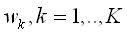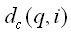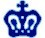EE6850 Home | Previsous: Features | Up: Intro |Next:Results

## CBIR #3: Combining Features and Making Decisions

This section formulates the problem of combining diferrent features as a problem of finding a set of weights of different feature distances, and then presents a Mini-Max algorithm in finding the best-matching image, and finally discusses the problem of relevance feedback as the adjustment of weights based on user input. Relevance feedback is shown to be degenerated with only 2 features, so it is not included in the current implementation.
______________________________________________

Mini-Max combination
Let's first look at a
more general case, where we have:
query image q, images in the database i,
K
features and thus K kinds of distances (they are constants)Assume we are going to combine them as a weighted sum of all the distances, i.e. the distance for a image in the database is written as:--(1)
and--(2).

Now we want to search for a vector w that satisfies Eq.(2) and the resulting distance measure is "most close" to our subjective criteria. There are two candidate approaches:
- assign a set of weights based on the perceptually judgement of the designer on some image set (training). But the problem here is that this set of weights may perform poorly on new dataset.
- or, having no assumption about the subjective judgement of a user, we choose the image that minimizes the maximum distance over all valid set of weights as the best match(denoted as Mini-Max hereafter).
For every image i, searching for the maximum distance over the weight space turns our to be a linear program, an thus have fast solution:

Maximize: (1), Subject to (2).
where all ds are the constants andare unknown.

The the image with the miminum "max-distance" is declared as the best match to the query image. Mini-Max is used in the current implementation, with the hope that it will generalized better to unknown dataset, and that the result will improve upon relevance feedback.(note1)

Relevance Feedback
Formulation:
- We obtain a set of images labelled "correct", and a set of images labelled "incorrect" from user input. Denote as the "+" class and the "-" class, respectively.
- The task of relevance feedback is to choose a set of weights that maximize the seperabiliy of the "+" class and the "-" class.
Define the seperabiliy between the two classes as the sum of all distances from one class to the other:-- (3)
Then this problem again becomes a linear program about:

Maximize: (3), Subject to (2).
where all ds are the constants
andare unknown. (note2)

Our case
From the discussion above, we can see feature combination and relevance feedback are linear programming problems that have fast solution
.
For our simple 2-feature case, the linear program degenerates to a 1-d function:
Color histogram distance, Edge histogram distance. Both lies in the range [0,1].
The max distanceof every image i, is a linear funtion of w over [0,1]. Thus the maximum either lies at w=0 or w=1, and comparingandis sufficient.
Then we rank the maximum ofandfor all i, and take n images with the least distance as our return result.
We can see that relevance feedback in 2-feature case, is comparing the 2 distance values of the "+" class to the "-" class at w=0 or w=1, and take the one with a larger D. This degeneracy make the problem less interesting, so we choose not to implement this module.

* We choose the Mini-Max criteria because it performs better than fixed weights in some of our experiments.Needless to say, this criteria also has many questionable assumptions, and sometimes it might be worse:
1. Mini-max is sensitive to outliers, so it tends to break if feature dimension is large. Taking the median may be a better idea in that case (median=mean for 2 features)
2. It will also break if some feature distance are biased, for example, if all edge distances lies in [0.9,1] but color distances are uniform on [0,1], then edge distance will essentially dominate the distance measure. A proper approach is to equalize the distances prior to combining them, although this doesn't seem to bother us in this HW.
3. We have little idea if feature space is uniform w.r.t. human perception.

** I'm sure people have better ideas of doing relevance feedback but I haven't checked the literature, too bad our simplistic formulation didn't work out interestingly.

 HW3 Intro System Features Decision Results Summary Appendix
Last update: 11/15/2001 7:49 PM